Quandaries and Queries Who is asking: Parent Level: Secondary Question: I have a 6 digit answer that is comprised of only 4 symbols: a square, a circle, a triangle, and an X. How many possible solutions are there and how is the best way to find out what ALL the solutions are? Hi Angela, By a "six digit answer" I expect you mean something like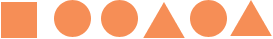or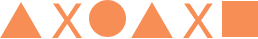Think about constructing such an answer. You have six positions to fill, starting from the left and working right. For the first position you can choose any of the four symbols, hence there are four, "one digit answers".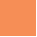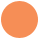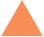and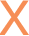Now add a second digit. Each of the four "one digit answers" can be extended to a "two digit answer" in four ways. For example the first "one digit answer" can be extended to the four "two digit answers",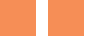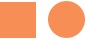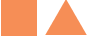and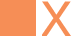In a similar way each of the "one digit answers" can be extended to four, "two digit answers". Hence there are 44 = 42 = 16 possible "two digit answers". In a similar way each of the 42 "two digit answers" can be extended to four "three digit answers", and hence there are 444 = 43 = 64 possible "three digit answers". You can complete the construction to see how many possible "six digit answers" there are. Penny Go to Math Central